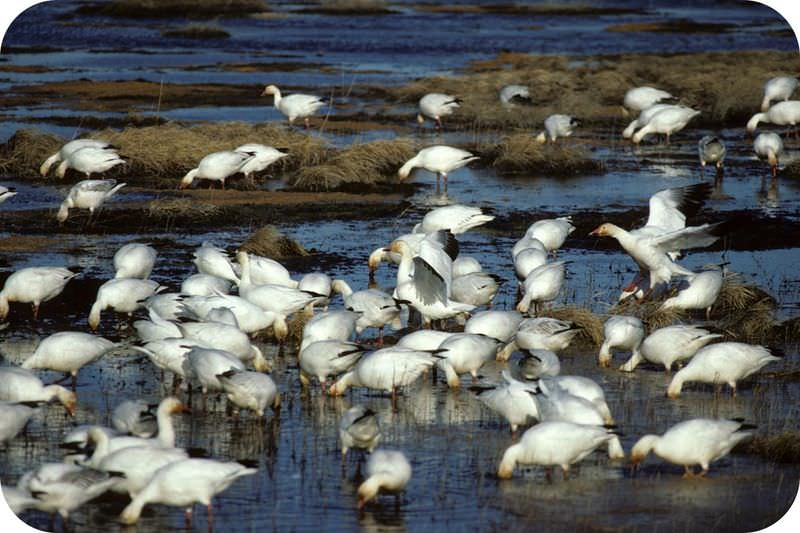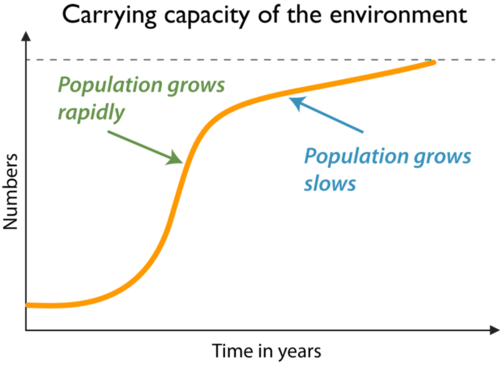# 17.6: Population Size

•• Contributed by CK12
• CK12How many geese are the right number for this area?

As many as can survive and have healthy offspring! The figure above shows a flock of snow geese at the Kenai National Wildlife Refuge. Their population will tend to grow as big as it can for the resources available. Once it is too large, some of its members will die off. This keeps the population size at the right number.

## How Populations Grow

A population usually grows when it has what it needs. If there’s plenty of food and other resources, the population will get bigger. Refer to the table below (Table below); it shows how a population of bacteria grew. A single bacteria cell was added to a container of nutrients. Conditions were ideal. The bacteria divided every 30 minutes. After just 10 hours, there were more than a million bacteria! Assume the bacteria population keeps growing at this rate. How many bacteria will there be at 10.5 hours? Or at 12 hours?

Time (hours) Number of Bacteria
0 1
0.5 2
1.0 4
1.5 8
2.0 16
2.5 32
3.0 64
3.5 128
4.0 256
4.5 512
5.0 1,024
5.5 2,048
6.0 4,096
6.5 8,192
7.0 16,384
7.5 32,768
8.0 65,536
8.5 131,072
9.0 262,144
9.5 524,288
10 1,048,576

Population Growth Rate

The population growth rate is how fast a population is growing. The letter r stands for the growth rate. The growth rate equals the number of new members added to the population in a year for each 100 members already in the population. The growth rate includes new members added to the population and old members removed from the population. Births add new members to the population. Deaths remove members from the population. The formula for population growth rate is:

r = b - d, where

b = birth rate (number of births in 1 year per 100 population members)
d = death rate (number of deaths in 1 year per 100 population members)

If the birth rate is greater than the death rate, r is positive. This means that the population is growing bigger. For example, if b = 10 and d = 8, r = 2. This means that the population is growing by 2 individuals per year for every 100 members of the population. This may not sound like much, but it’s a fairly high rate of growth. A population growing at this rate would double in size in just 35 years!

If the birth rate is less than the death rate, r is negative. This means that the population is becoming smaller. What do you think might cause this to happen?

Carrying Capacity

A population can’t keep growing bigger and bigger forever. Sooner or later, it will run out of things it needs. For a given species, there is a maximum population that can be supported by the environment. This maximum is called the carrying capacity. When a population gets close to the carrying capacity, it usually grows more slowly (Figure below). When the population reaches the carrying capacity, it stops growing.A population can’t get much larger than the carrying capacity. What might happen if it did?

## Summary

• A population will grow as long as it has what it needs.
• The carrying capacity of an environment is reached when the number of births equal the number of deaths.
• A limiting factor determines the carrying capacity for a species.

## Review

1. What happens if a population exceeds its carrying capacity?
2. Explain how a single bacterium can grow to a population of more than a million 10 hours later.
3. What happens if the birth rate in a population is more than the death rate? What happens if it is less?